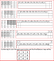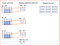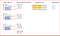# Convolutional Neural Network(CNN) Simplified

Interested in understanding how some of the apps today classify images of your family and friends and have basics of machine learning then this is the article for you.

Let’s first understand how our brain recognizes objects. We will learn what CNN is, how CNN uses the motivation from brain for object recognition and how CNN works.

Let’s understand how our brain recognizes an image

According to Hubel and Wiesel, Nobel Prize winning professors, the visual area V1 consists of simple cells and complex cells. Simple cells helps with feature detection and complex cells combine several such local features from small spatial neighborhood. Spatial pooling helps with translational invariant features.

When we see a new image, we scan the image may be left to right and top to bottom to understand the different features of the image. Our next step is combine the different local features that we scanned to classify the image. . This is exactly how CNN works

what does translational invariant features mean?

Invariance of image implies that even when an image is rotated, sized differently or viewed in different illumination an object will be recognized as the same object.

This helps with object recognition as the image representation is invariant to image transformations such as translation, rotation, or small deformations etc.

we use convolution neural networks for image recognition and classification.

Let’s understand what is CNN and how can we use it.

# What is CNN?

CNN stands for Convolutional Neural Network which is a specialized neural network for processing data that has an input shape like a 2D matrix like images.

CNN’s are typically used for image detection and classification. Images are 2D matrix of pixels on which we run CNN to either recognize the image or to classify the image. Identify if an image is of a human being, or car or just digits on an address.

Like Neural Networks, CNN also draws motivation from brain . We use object recognition model proposed by Hubel and Wiesel.

## What is convolution?

Convolution is a mathematical operation where we have an input I, and an argument, kernel K to produce an output that expresses how the shape of one is modified by another.

let’s explain in terms of an image.

We have an image “x”, which is a 2D array of pixels with different color channels(Red,Green and Blue-RGB) and we have a feature detector or kernel “w” then the output we get after applying a mathematical operation is called a feature map

The mathematical operation helps compute similarity of two signals.

we may have a feature detector or filter for identifying edges in the image, so convolution operation will help us identify the edges in the image when we use such a filter on the image.

we usually assume that convolution functions are zero everywhere but the ﬁnite set of points for which we store the values. This means that in practice we can implement the inﬁnite summation as a summation over a ﬁnite number of array elements.

Since convolution is commutative, we can rewrite the equation pictured above as shown below. We do this for ease of implementation in Machine Learning, as there is less variation in range of valid values for m and n. This is cross correlation function which most neural networks use.

So, how do we implement that in CNN?

The way we implement this is through Convolutional Layer

Convolutional layer is core building block of CNN, it helps with feature detection.

Kernel K is a set of learnable filters and is small spatially compared to the image but extends through the full depth of the input image.

An easy way to understand this is if you were a detective and you are came across a large image or a picture in dark, how will you identify the image?

You will use you flashlight and scan across the entire image. This is exactly what we do in convolutional layer.

Kernel K, which is a feature detector is equivalent of the flashlight on image I, and we are trying to detect feature and create multiple feature maps to help us identify or classify the image.

we have multiple feature detector to help with things like edge detection, identifying different shapes, bends or different colors etc.

How does this all work?

let’s take an image of 5 by 5 matrix with 3 channels(RGB) , a feature detector of 3 by 3 with 3 channels (RGB) and scan the feature detector over the image by 1 stride.

What will be the dimension of the output matrix or feature map when I apply a feature detector over an image?

Dimension of the feature map as a function of the input image size(W), feature detector size(F), Stride(S) and Zero Padding on image(P) is

(W−F+2P)/S+1

Input image size W in our case is 5.

Feature detector or receptive field size is F, which in our case is 3

Stride (S) is 1, and the amount of zero padding used (P) on the image is 0.

so, our feature map dimension will (5–3 +0)/1 + 1=3.

so feature map will a 3*3 matrix with three channels(RGB).

This is explained step by step below aFeature map based on the input image and feature detector using cross correlation function.

We see that 5 by 5 input image is reduced to 3 by 3 feature maps. The depth or channels remain the same as 3(RGB)

we use multiple feature detectors for finding edges, we can use feature detector to sharpen the image or to blur the image.

If we do not want to reduce the feature map dimension then we can use zero padding of one as shown below

in that case applying the same formula, we get

(W−F+2P)/S+1 => (5–3 +2)/1 + 1=5,

now the dimension of output will be 5 by 5 with 3 color channels(RGB).

Let’s see all this in action

If we have one feature detector or filter of 3 by 3, one bias unit then we first apply linear transformation as shown below

output= input*weight + biasInput image of 5 by 5 with the three color channels and a feature detector or filter of 3 by 3 with a bias unit and stride is 1

No. of parameters = (3 * 3 * 3 )+1 = 28

For 100 feature detectors or filters, number of parameters will 2800.

After every convolution operation which is a linear function, we apply ReLU activation function. ReLU activation function introduces non linearity in convolutional layer.

It replaces all negative pixel values with zero values in the feature map.

Below figure shows the feature map transformation after applying the ReLU activation function.

Now that we have completed the feature detection from local areas we will combine all such feature detection from spatial neighborhood to build the picture.

Remember you are a detective scanning an image in dark, you have now scanned the image from left to right and top to bottom. now we need to combine all the feature to recognize the image

# Pooling

we now apply pooling to have translational invariance. (remember the rose image)

Invariance to translation means that when we change the input by a small amount the pooled outputs do not change. This helps with detecting features that are common in the input like edges in an image or colors in an image

We apply the max pooling function which provides a better performance compared to min or average pooling.

when we use max pooling it summarizes the output over a whole neighborhood. we now have fewer units compared to the feature map.

In our example, we scan over all the feature maps using a 2 by 2 box and find the maximum value.Applying max pooling to the output using a 2 by 2 box. Highlighted region in yellow has a max value of 6

so now we know that a convolutional network consists of

• Multiple convolutions performed in parallel — output is linear activation function
• Applying nonlinear function ReLU to the convolutional layers
• Use a pooling function like max pooling to summarize the statistics of nearby locations. This helps with “Translational Invariance
• we flatten the max pooled output which are then inputs to a fully connected neural network

Below diagram is the full convolutional neural network

Is there a reason to use convolution for image detection?

## Convolution uses three important ideas

• Sparse interactions
• Parameter sharing
• Equivariant representations

Sparse interaction or sparse weights is implemented by using kernels or feature detector smaller than the input image.

If we have an input image of the size 256 by 256 then it becomes difficult to detect edges in the image may occupy only a smaller subset of pixels in the image. If we use smaller feature detectors then we can easily identify the edges as we focus on the local feature identification.

one more advantage is computing output requires fewer operations making it statistically efficient.

Parameter Sharing is used to control the number of parameters or weights used in CNN.

In traditional neural networks each weight is used exactly once however in CNN we assume that if the one feature detector is useful to compute one spatial position then it can be used to compute a different spatial position.

As we share parameters across the CNN, it reduces the number of parameters to be learnt and also reduces the computational needs.

Equivariant representation

It means that object detection is invariant to the changes in illumination, change of position, but internal representation is equivariance to these changes

represent(rose) = represent(transform(rose)).

## Data Driven Investor

### By Data Driven Investor

In each issue we share the best stories from the Data-Driven Investor's expert community. Take a look

Written by

Written by

## Renu Khandelwal

#### Loves learning, sharing, and discovering myself. Passionate about Machine Learning and Deep Learning